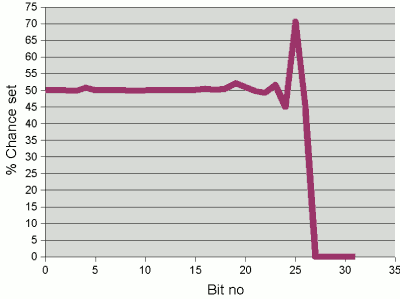# How the String hash function works (2)

## Ironing out the bias

This page continuous from page 1 of our discussion on how the Java String hash function works. So far, we posed a hypothetical set of random strings, where each character has particular distribution. We found that, as is actually quite typical with strings, characters are biased towards certain bits being set. In our example, bits 4 and 5 had a just over 70% and 75% chance of being set respectively for a given character. The lower bits showed better randomness: i.e. their chance of being set was closer to 50%.

The trick of the string hash function is to try and iron out this bias by mixing bits with a biased probability with other bits, in particular those where the probability is already fairly unbiased.

### First: a not-so-good hash function

Recall that the Java String function combines successive characters by multiplying the current hash by 31 and then adding on the new character. To show why this works reasonably well for typical strings, let's start by modifying it so that we instead use the value 32 as the hash code. In other words, let's imagine the hash function is this:

 ```int hash = 0; for (int i = 0; i < length(); i++) { hash = 32 * hash + charAt(i); } return hash; ``` Note that this is a arguably bad hash function for strings1 (and possibly for most things). The problem is that we are multiplying by a power of 2, which in effect simply shifts the current hash along. Some mixing then does take place: the high bits of the ith character will be mixed with the lower bits of the previous character (now shifted left 5 places so that its lower 3 bits coincide with the upper 3 bits of the ith character). However, this isn't enough to completely remove the pattern of biases from the resulting hash code. The problem can be illustrated if we take random series of 5 characters (with the distribution we've been discussing). The graph to the right shows the probability of each successive bit of the hash code being set. The graph still has characteristic "humps" where the bits with high probability in the original characters making up the hash code don't get enough chance to interact with more randomly distributed bits. (Although the "hump" does now span a width of 1 bit rather than 2 in the original distribution.)Probability of successive bits being set in the hash code of a randomly-generated 5 character string, when 32 is chosen as the multiplier in the hash function.

### Improving the multiplier

Now we plot the same graph using 31 as the multiplier, as in the Java implementation of the String hash code function. Multiplying by 31 effectively means that we are shifting the hash by 5 places but then subtracting the original unshifted value:

```int hash = 0;
for (int i = 0; i < length(); i++) {
hash = (hash << 5) - hash + charAt(i);
}
return hash;
```

There are now further interaction among the bits. Firstly, adding the ith character and subtracting the hash (with the previous character in its lower bits) brings the per-bit probabilities in the character closer to 50%. (For example, if we have two bits, each with a probability of 70% of being set, then subtracting one from the other leaves a probability of 42% that the resulting bit will be set, with a 21% chance of there being a carry from the bit to the left.) And now, rather than just combining the high bits of the ith number with the low bits of the previous character, there are interactions generally between the lower bits of the nth character and the higher bits of the n-1th character.Probability of successive bits being set in the hash code of a randomly-generated 5 character string, when 31 is chosen as the multiplier in the hash function.

The result of these various points of interaction is a much flatter bit distribution in the restulting hash code. With 5 character keys, the profile is fairly flat up to bit 22, meaning that we can store a few million such keys fairly efficiently.

## Next...

Depending on your needs, here are some suggestions of what to read next:

1. It is occasionally given as a string hash function, e.g. in Granet, V. (2004), Algorithmique et programmation en Java, Dunod, 2nd ed, p. 277, but if suitable, this is only when the hash table has a prime number of buckets (which the HashMap implementation no longer does).

If you enjoy this Java programming article, please share with friends and colleagues. Follow the author on Twitter for the latest news and rants.